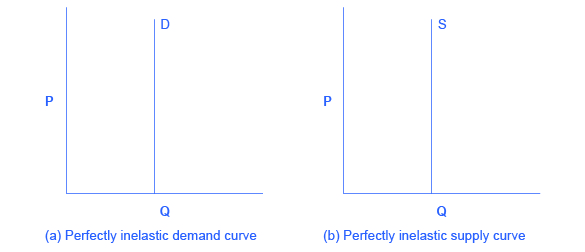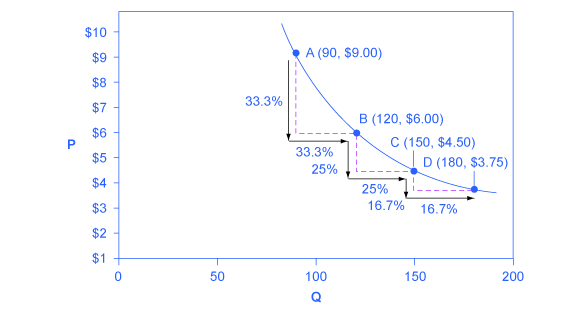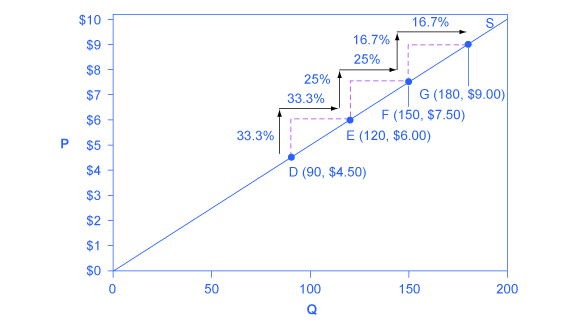Material Type:
Module
Provider:
Rice University
Tags:
Constant Unitary Elasticity, Demand Curve, Infinite Elasticity, Perfect Elasticity, Perfect Inelasticity, Zero Inelasticity
Language:
English

# Polar Cases of Elasticity and Constant Elasticity

## Overview

By the end of this section, you will be able to:

• Differentiate between infinite and zero elasticity
• Analyze graphs in order to classify elasticity as constant unitary, infinite, or zero

There are two extreme cases of elasticity: when elasticity equals zero and when it is infinite. A third case is that of constant unitary elasticity. We will describe each case. Infinite elasticity or perfect elasticity refers to the extreme case where either the quantity demanded (Qd) or supplied (Qs) changes by an infinite amount in response to any change in price at all. In both cases, the supply and the demand curve are horizontal as Figure shows. While perfectly elastic supply curves are for the most part unrealistic, goods with readily available inputs and whose production can easily expand will feature highly elastic supply curves. Examples include pizza, bread, books, and pencils. Similarly, perfectly elastic demand is an extreme example. However, luxury goods, items that take a large share of individuals’ income, and goods with many substitutes are likely to have highly elastic demand curves. Examples of such goods are Caribbean cruises and sports vehicles.Infinite Elasticity The horizontal lines show that an infinite quantity will be demanded or supplied at a specific price. This illustrates the cases of a perfectly (or infinitely) elastic demand curve and supply curve. The quantity supplied or demanded is extremely responsive to price changes, moving from zero for prices close to P to infinite when prices reach P.

Zero elasticity or perfect inelasticity, as Figure depicts, refers to the extreme case in which a percentage change in price, no matter how large, results in zero change in quantity. While a perfectly inelastic supply is an extreme example, goods with limited supply of inputs are likely to feature highly inelastic supply curves. Examples include diamond rings or housing in prime locations such as apartments facing Central Park in New York City. Similarly, while perfectly inelastic demand is an extreme case, necessities with no close substitutes are likely to have highly inelastic demand curves. This is the case of life-saving drugs and gasoline.Zero Elasticity The vertical supply curve and vertical demand curve show that there will be zero percentage change in quantity (a) demanded or (b) supplied, regardless of the price.

Constant unitary elasticity, in either a supply or demand curve, occurs when a price change of one percent results in a quantity change of one percent. Figure shows a demand curve with constant unit elasticity. Constant unitary elasticity, in either a supply or demand curve, occurs when a price change of one percent results in a quantity change of one percent. Figure 5.6 shows a demand curve with constant unit elasticity. Using the midpoint method, you can calculate that between points A and B on the demand curve, the price changes by 28.6% and quantity demanded also changes by 28.6%. Hence, the elasticity equals 1. Between points B and C, price again changes by 28.6% as does quantity, while between points C and D the corresponding percentage changes are 22.2% for both price and quantity. In each case, then, the percentage change in price equals the percentage change in quantity, and consequently elasticity equals 1. Notice that in absolute value, the declines in price, as you step down the demand curve, are not identical. Instead, the price falls by $2.00 from A to B, by a smaller amount of$1.50 from B to C, and by a still smaller amount of $0.90 from C to D. As a result, a demand curve with constant unitary elasticity moves from a steeper slope on the left and a flatter slope on the right—and a curved shape overall. Notice that in absolute value, the declines in price, as you step down the demand curve, are not identical. Instead, the price falls by$23 from A to B, by a smaller amount of $1.50 from B to C, and by a still smaller amount of$.90 from C to D. As a result, a demand curve with constant unitary elasticity has a steeper slope on the left and a flatter slope on the right—and a curved shape overall.A Constant Unitary Elasticity Demand Curve A demand curve with constant unitary elasticity will be a curved line. Notice how price and quantity demanded change by an identical percentage amount between each pair of points on the demand curve.

Unlike the demand curve with unitary elasticity, the supply curve with unitary elasticity is represented by a straight line, and that line goes through the origin. In each pair of points on the supply curve there is an equal difference in quantity of 30. However, in percentage value, using the midpoint method, the steps are decreasing as one moves from left to right, from 28.6% to 22.2% to 18.2%, because the quantity points in each percentage calculation are getting increasingly larger, which expands the denominator in the elasticity calculation of the percentage change in quantity.

Consider the price changes moving up the supply curve in Figure. From points D to E to F and to G on the supply curve, each step of \$1.50 is the same in absolute value. However, if we measure the price changes in percentage change terms, using the midpoint method, they are also decreasing, from 28.6% to 22.2% to 18.2%, because the original price points in each percentage calculation are getting increasingly larger in value, increasing the denominator in the calculation of the percentage change in price. Along the constant unitary elasticity supply curve, the percentage quantity increases on the horizontal axis exactly match the percentage price increases on the vertical axis—so this supply curve has a constant unitary elasticity at all points.A Constant Unitary Elasticity Supply Curve A constant unitary elasticity supply curve is a straight line reaching up from the origin. Between each pair of points, the percentage increase in quantity supplied is the same as the percentage increase in price.

# Key Concepts and Summary

Infinite or perfect elasticity refers to the extreme case where either the quantity demanded or supplied changes by an infinite amount in response to any change in price at all. Zero elasticity refers to the extreme case in which a percentage change in price, no matter how large, results in zero change in quantity. Constant unitary elasticity in either a supply or demand curve refers to a situation where a price change of one percent results in a quantity change of one percent.

# Self-Check Questions

Why is the demand curve with constant unitary elasticity concave?

## Hint:

The demand curve with constant unitary elasticity is concave because the absolute value of declines in price are not identical. The left side of the curve starts with high prices, and then price falls by smaller amounts as it goes down toward the right side. This results in a slope of demand that is steeper on the left but flatter on the right, creating a curved, concave shape.

Why is the supply curve with constant unitary elasticity a straight line?

## Hint:

The constant unitary elasticity is a straight line because the curve slopes upward and both price and quantity are increasing proportionally.

# Review Questions

Describe the general appearance of a demand or a supply curve with zero elasticity.

Describe the general appearance of a demand or a supply curve with infinite elasticity.

# Critical Thinking Question

Can you think of an industry (or product) with near infinite elasticity of supply in the short term? That is, what is an industry that could increase Qs almost without limit in response to an increase in the price?

# Problems

The supply of paintings by Leonardo Da Vinci, who painted the Mona Lisa and The Last Supper and died in 1519, is highly inelastic. Sketch a supply and demand diagram, paying attention to the appropriate elasticities, to illustrate that demand for these paintings will determine the price.

Say that a certain stadium for professional football has 70,000 seats. What is the shape of the supply curve for tickets to football games at that stadium? Explain.

When someone’s kidneys fail, the person needs to have medical treatment with a dialysis machine (unless or until they receive a kidney transplant) or they will die. Sketch a supply and demand diagram, paying attention to the appropriate elasticities, to illustrate that the supply of such dialysis machines will primarily determine the price.